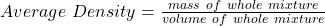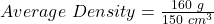Question

100 cm cubic of water is mixed with 50 centimetres cubic of concentrated acid of density 1.2 gram per centimetre cube assuming no change in volume find the average density of the mixture the density of water is 1.0 gram per centimetre cube​

1.Average Density = 1.07 g/cm³

Explanation:

The average density of the whole mixture is given by the following formula:where,

volume of whole mixture = volume of water + volume of acid

volume of whole mixture = 100 cm³ + 50 cm³ = 150cm³

mass of whole mixture = mass of water + mass of acid

mass of whole mixture = (volume of water)(density of water) + (volume of acid)(density of acid)

mass of whole mixture = (100 cm³)(1 g/cm³) + (50 cm³)(1.2 g/cm³)

mass of whole mixture = 160 g

Therefore,Average Density = 1.07 g/cm³